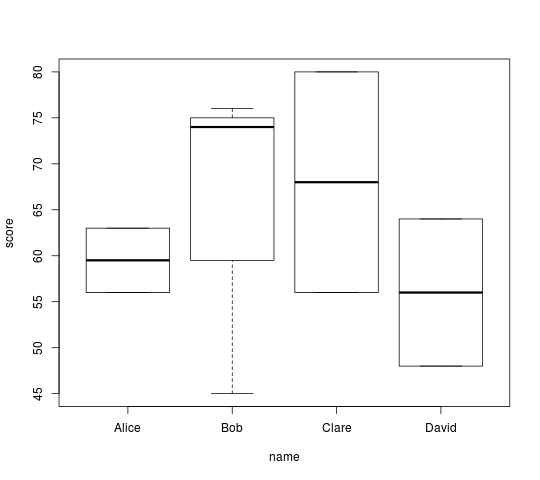# Introducing R in three lines

## Overview

R is an environment for analysing and visualising data, as well as being a programming language, but it is much more than just that. The following is intended to give a brief flavour of R and to introduce some of its key features.

## Read, summarise and plot data in 3 short lines of R code

### The input data

Here is a simple 2 column data file with some (made up) exam results for 4 students:

```name    score
Alice   56
Bob     76
Clare   80
David   48
Bob     45
Bob     74
Alice   63
Clare   56
David   64
```

First of all we read the data into R:

```results <- read.table("results.dat", header = TRUE)
```

R automatically picks up the names of each column from the file (via "`header=TRUE`"). Unlike many programming languages, there is no need to loop explicitly over each row of data. Neither do we need to specify data types or declare the object "`results`" which stores the data in R. However, R provides fine-grained control of such details should you want it (see "`?read.table`" within R for the relevant help page).

In this case, "`results`" is a data frame, which is a very common data structure in R, resembling a table or spreadsheet of rows and columns of values, with identical data types in each column.

### Summarise the data

Having read in the data, we might want to calculate the mean score for each student. We can do this easily, using the "`aggregate`" function:

```aggregate(score ~ name, data = results, FUN = mean)
```
```   name score
1 Alice  59.5
2   Bob  65.0
3 Clare  68.0
4 David  56.0
```

Note here the use of R's formula syntax ("`~`") for clearly and concisely expressing that we want to perform some action on the variable "`score`", according to the different values in the corresponding variable "`name`"; both of these variables are to be found in the object "`results`".

In this case, we want to calculate the mean value of "`score`" for each `name`, but you can supply any suitable function to the argument "`FUN`", such as `sum`, `min`, `max` etc. or you can write your own function to use.

### Visualise the data

In addition to summarising the data on screen, you typically also want to plot the results as a graph. There are different ways to do this, but R assigns a default style of graph according to the type of object being plotted, in accordance with its object oriented design:

```plot(results)
```The first 2 columns of the data frame "`results`" are automatically used for the `X` and `Y` axis data for plotting. Since the "`names`" column is not numeric data, R reads it in as a factor by default and correspondingly produces a boxplot summary of the results for each student.

Once again, fine control over the plot is achieved with further header arguments to "`plot`"; see "`?boxplot`" and "`?par`" for the relevant help pages.

You could plot the same graph explicitly, using the formula syntax from above, as follows:

```plot(score ~ name, data = results)
```

You can find out more about object orientation in R from these help pages: "`?class`" and "`?S3Methods`". For example, try the following (anything after a "`#`" is treated as a comment and ignored by R):

```class(results)                  # "data.frame"
methods(class = "data.frame")
```

## Summary

So, there you have it, a quick introduction to R in only 3 lines of code!

```results <- read.table("results.dat", header = TRUE)
aggregate(score ~ name, data = results, FUN = mean)
plot(results)
```

## Key concepts introduced

• emphasizes the difference between a programming language and a language for computing with data
• R has a concise, intuitive and readily customisable syntax: it enables fine-grained control, but with sensible default choices.
• The data-driven design of R avoids the need to explicitly loop over vector/array elements, declare variables or specify data types etc. (although all these aspects are under user-control).
• use of (well-chosen) column names avoids ambiguity, plus is much more readable and easy to follow (i.e. no references to, e.g. "column 2")
• demonstrates the powerful formula interface, which is central to building models and manipulating hierarchical data structures in R
• introduces data frames: the key data structure in R (see "`?data.frame`")
• introduces factors, an efficient format for categorical data (see "`?factor`")
• introduces the "everything is an object" approach: "`mean`" is passed as a function not as a character string; any other appropriate function could be used instead
• R is object oriented (plot method), i.e. different "methods" produce different results, according to the "`class`" of the object on which they operate.
• R supports and encourages a structured approach to data analysis.